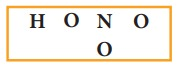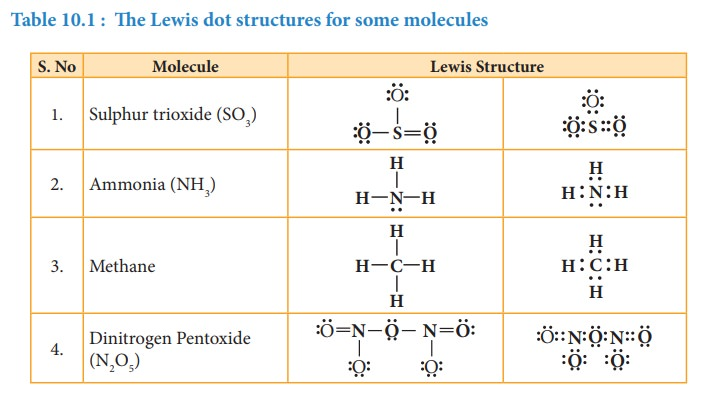Home | | Chemistry 11th std | Representing a covalent bond - Lewis structure (Lewis dot structure)

# Representing a covalent bond - Lewis structure (Lewis dot structure)

Lewis structure (Lewis dot structure) is a pictorial representation of covalent bonding between the combining atoms.

Representing a covalent bond - Lewis structure (Lewis dot structure)

Lewis structure (Lewis dot structure) is a pictorial representation of covalent bonding between the combining atoms. In this structure the shared valence electrons are represented as a pair of dots between the combining atoms and the unshared electrons of the atoms are represented as a pair of dots (lone pair) on the respective individual atoms.

The Lewis dot structure for a given compound can be written by following the steps given below. Let us understand these steps by writing the Lewis structure for water.

1. Draw the skeletal structure of the molecule. In general, the most electronegative atom is placed at the centre. Hydrogen and fluorine atoms should be placed at the terminal positions. For water, the skeletal structure is2. Calculate the total number of valence electrons of all the atoms in the molecule. In case of polyatomic ions the charge on ion should also be considered during the calculation of the total number of valence electrons. In case of anions the number of negative charges should be added to the number of valence electrons. For positive ions the total number of positive charges should be subtracted from the total number of valence electrons.

In water, total number of valence electron = [2×1 (valence electron of hydrogen)] + [1 × 6 (valence electrons of oxygen)] = 2 + 6 = 8.3. Draw a single bond between the atoms in the skeletal structure of the molecule. Each bond will account for two valence electrons (a bond pair). For water, we can draw two bonds accounting for four valence electrons as follows.4. Distribute the remaining valence electrons as pairs (lone pair), giving octet (only duet for hydrogen) to the atoms in the molecule. The distribution of lone pairs starts with the most electronegative atoms followed by other atoms.

In case of water, the remaining four electrons (two lone pairs) are placed on the most electronegative central oxygen, giving octet.5. Verify weather all the atoms satisfy the octet rule (for hydrogen duet). If not, use the lone pairs of electrons to form additional bond to satisfy the octet rule.

In case of water, oxygen has octet and the hydrogens have duets, hence there is no need for shifting the lone pairs. The Lewis structure of water is as followsLet us draw the Lewis structure for nitric acid.

1. Skeletal structure2. Total number of valence electrons in HNO3

= [1 × 1(hydrogen)] + [1 × 5(nitrogen)] + [3× 6(oxygen)] = 1+ 5 + 18 = 24

3. Draw single bonds between atoms. Four bonds can be drawn as shown in the figure for HNO3 which account for eight electrons (4 bond pairs).4. Distribute the remaining sixteen (24 - 8= 16) electrons as eight lone pairs starting from most electronegative atom, the oxygen. Six lone pairs are distributed to the two terminal oxygens (three each) to satisfy their octet and two pairs are distributed to the oxygen that is connected to hydrogen to satisfy its octet.5. Verify weather all the atoms have octet configuration. In the above distribution, the nitrogen has one pair short for octet. Therefore, move one of the lone pair from the terminal oxygen to form another bond with nitrogen.

The Lewis structure of nitric acid is given asStudy Material, Lecturing Notes, Assignment, Reference, Wiki description explanation, brief detail
11th Chemistry : UNIT 10 : Chemical bonding : Representing a covalent bond - Lewis structure (Lewis dot structure) |